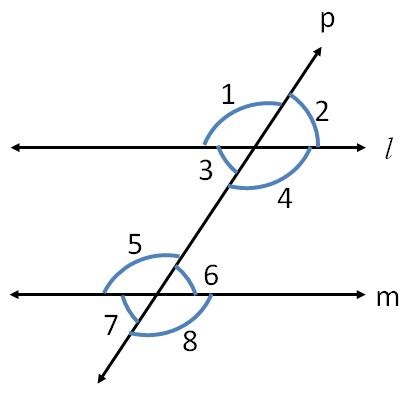Angles made by the transversal - Theory

Chapter 5 Class 7 Lines and Angles
Concept wise

Let us consider

two parallel lines l & m with transversal pHere, ∠1 to ∠8 are the angles formed by these lines.

Let’s learn what they are.

### Transcript

Let us consider two parallel lines l & m with transversal p Here, ∠1 to ∠8 are the angles formed by these lines. Let’s learn what they are.